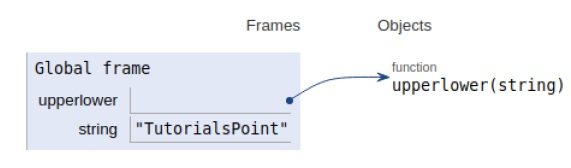# Count upper and lower case characters without using inbuilt functions in Python program

Problem statement − We are given a string, we need to count the number of uppercase and lowercase characters present in the string without using the inbuilt function

This can be easily solved by using islower() and isupper() function available in python. But here there is a constraint to use the inbuilt function. So here we take the help of the ASCII value of the characters.

Using the ord() function we compute the ASCII value of each character present in the string and then compare to check for uppercase and lowercase as shown below.

## Example

Live Demo

def upperlower(string):
upper = 0
lower = 0
for i in range(len(string)):
# For lowercase
if (ord(string[i]) >= 97 and
ord(string[i]) <= 122):
lower += 1
# For uppercase
elif (ord(string[i]) >= 65 and
ord(string[i]) <= 90):
upper += 1
print('Lower case characters = '+str(lower))
print('Upper case characters = '+str(upper))
# Driver Code
string = 'TutorialsPoint'
upperlower(string)

## Output

Lower case characters = 12
Upper case characters = 2All the variables are declared in the local scope and their references are seen in the figure above.

## Conclusion

In this article, we have learned how to count uppercase and lowercase characters present in the given string